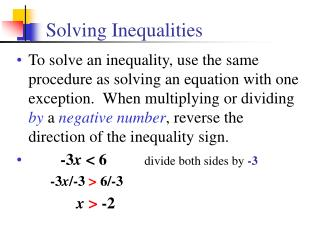DownloadDownload PresentationSolving Inequalities

# Solving Inequalities

Télécharger la présentation## Solving Inequalities

- - - - - - - - - - - - - - - - - - - - - - - - - - - E N D - - - - - - - - - - - - - - - - - - - - - - - - - - -
##### Presentation Transcript

1. Solving Inequalities • To solve an inequality, use the same procedure as solving an equation with one exception.When multiplying or dividing by a negative number, reverse the direction of the inequality sign. • -3x < 6divide both sides by -3 -3x/-3 > 6/-3 x> -2

2. -2 -1 0 Solving Inequalities • -4x + 2 > 10 -4x > 8 x < -2 • To graph the solution set, circle the boundary and shade according to the inequality. • Use an open circle for < or > and closed circles for ≤ or ≥.

3. 0 1 2 Solving Inequalities • 3b - 2(b - 5) < 2(b + 4) 3b - 2b + 10 < 2b + 8 b + 10 < 2b + 8 -b + 10 < 8 -b < -2 b > 2

4. Solving Absolute Value Inequalities • Solving absolute value inequalities is a combination of solving absolute value equations and inequalities. • Rewrite the absolute value inequality. • For the first equation, all you have to do is drop the absolute value bars. • For the second equation, you have to negate the right side of the inequality and reverse the inequality sign.

5. 2x + 4 > 12 or 2x + 4 < -12 2x > 8 2x < -16 x > 4 or x < -8 x < -8 or x > 4 4 -8 0 Solve: |2x + 4| > 12

6. 9 -1 0 Solve: 2|4 - x| < 10 |4 - x| < 5 4 - x < 5 and 4 - x> -5 - x < 1 - x > -9 x > -1 and x < 9 -1 < x < 9